## Example Questions

2 Next →

### Example Question #1921 : Sat Mathematics

Solve for: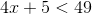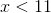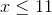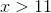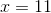Explanation:

We want to isolate the variable on one side and numbers on another side. Treat like a normal equation.Subtracton both sides.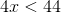Divideon both sides.### Example Question #11 : How To Find The Solution To An Inequality With Addition

Solve for: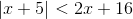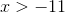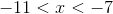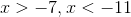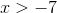Explanation:

We want to isolate the variable on one side and numbers on another side. Treat like a normal equation.We need to set-up two equations since it's absolute value.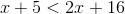Subtract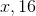on both sides.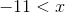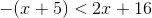Distribute the negative sign to each term in the parenthesis.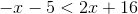Addand subtracton both sides.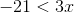Divideon both sides.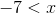We must check each answer. Let's try.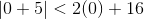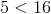This is true thereforeis a correct answer. Let's next try.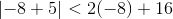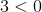This is not true thereforeis not correct.

Final answer is just.

2 Next →

### All SAT Math Resources